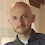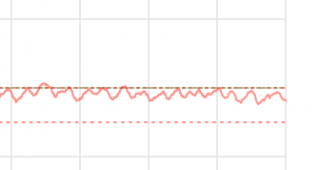## 2018-08-25

### E=mc²

One of the iconic equations in science, E=mc², but it seems a tad convenient to me.

Essentially, as c is a constant, it is just E=qm where q is a constant. I.e. Energy and mass have an conversion factor that is simply a constant number.

But if we did not measure any of the units of energy, mass, distance or time in the exact units we do (and most of those are totally arbitrary units) it would be a different constant, and not exactly c²

So why is the equation so conveniently E=mc² and not E=qmc² or E=qm ?

And yes, I got an A in physics O'level, but it was a long time ago...

1.Not sure I understand why any of those are more convenient than any of the others, but as far as the units are concerned, while arbitrary they are also very much linked:

2.Physicists often treat standard constants as 1, as they are tied to our real-world measurement units for real-world use but can be anything in the abstract. So in their case E = m, and they often don't even bother with m - it's all energy anyway.

3.That’s an interesting question. I think there’s a clue when you said that MOST of the units are totally arbitrary. The SI system is what’s called a “coherent” system of units, which means that the units are defined in relation to each other according to the physical laws and with no multiplication factors (so 1 joule of energy is defined as 1 kg m^2 s^-2). And one of the the advantages of doing things that way is that it simplifies the equations, so we can say that e = mc^2 in any coherent system of units. In an incoherent system of units there would be a conversion factor, as you say.

4.So, my first comment has not shown up. I'm hopeing that was because Adrian didn't see it rather than he censored it.

Joules is a derived unit so any change in the definition of the metre, second or kilogramme will change the size of a Joule.

5.It would still be C squared, in whatever units you were using. But the measurement of energy would then be in KgFeet2/minute 2 instead.

6.As others have said, you can use any units you want as long as you're consistent in your derivation of energy (ie that unit is not "totlly arbitrary"; it comes from the others).

It's like saying "In the UK we count using ten digits and my keyboard just happens to have them all on there. If we used something other than ten digits then the keyboard would have to be different, yet it isn't. I find that a bit convenient."

PBC Space Time's vid on the origin and meaning of this equation is interesting.

7.the e stands for eggs

8.The key to understanding this is Dimensional Analysis. This is where we break down quantities into their base units. All quantities can be expressed as combinations of the four elements, Length (L), Mass (M), Time (T), and Electric Charge (C). So the speed of light has dimensions L/T Length divided by time.

The kinetic energy of a body is the its Mass x Velocity. So the formula says that the energy of matter is equivalent to its kinetic energy if it were traveling at the speed of light squared.

1.ke = ½mv².

So the actual hypothetical (non-relativistic) speed would be sqrt(2) times c.

I used to be able to derive E=mc² from first principles, but I've forgotten the details. Fortunately we have Wikipedia.

https://en.wikipedia.org/wiki/Mass%E2%80%93energy_equivalence#The_first_derivation_by_Einstein_(1905)

9.https://www.amazon.co.uk/Why-Does-mc2-Should-Care/dp/0306819112

is a good read and explains it all.

10.https://www.amazon.co.uk/Why-Does-mc2-Should-Care/dp/0306819112

is a good read and explains it all.

11.In advanced physics texts, very often it is chosen to let c=1 and define the system of units accordingly with also h=1 or h bar = 1. So then E = m.

The reason that it was E=mc² is because that is correct _in the particular system of units in use_, SI units and with a particular definition of ‘total relativistic energy’.

Comments are moderated purely to filter out obvious spam, but it means they may not show immediately.

### Setting the temperature

My air-con can have a temperature set and aim for it. It has a wide range of ± a few degrees which I don't like. It is not setting tempe...